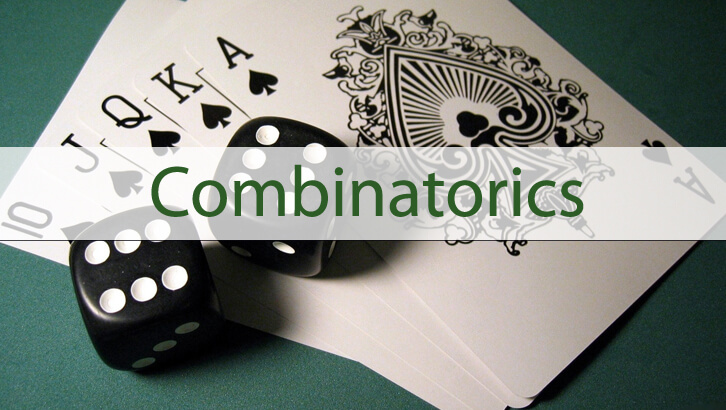# CAT Permutation Combination and Functions

Question

How many functions can be defined from Set A — {1, 2, 3, 4} to Set B = {a, b, c, d} that are neither one-one nor onto?

Explanation

To start with, if you do not know the meaning of one-one or onto, look these up. For good measure know the meanings of the terms surjective, injective etc also. CAT tests these terms.

Let us start by answering a far simpler question. How many functions are possible from Set A to Set B. This is equal to 4^4 = 256.

Note that any function from Set A to Set B that is one-one will also be onto and vice versa. How? Why? – Think about this. Remember, tutors can only take the horse to the pond. 🙂

So, we need to subtract only those functions that are one-one AND onto. Or, effectively we have to eliminate those functions where {1, 2, 3, 4} are mapped to {a, b, c, d} such that each is mapped to a different element. This is effectively same as the number of ways of rearranging 4 elements. Or, number of ways of doing this is 4! .

So, total number of functions that are neither one-one nor onto = 256 – 24 = 232.